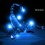An inequality

Today I met a prob:

"Prove that $(sin \alpha)^{2007} + cos \alpha < \frac{5}{4}$ (With $\alpha$ is an acute angle)

Please tell me the way to solve the prob. (or if the prob's wrong)Note by Đức Việt Lê
6 years, 4 months ago

This discussion board is a place to discuss our Daily Challenges and the math and science related to those challenges. Explanations are more than just a solution — they should explain the steps and thinking strategies that you used to obtain the solution. Comments should further the discussion of math and science.

When posting on Brilliant:

• Use the emojis to react to an explanation, whether you're congratulating a job well done , or just really confused .
• Ask specific questions about the challenge or the steps in somebody's explanation. Well-posed questions can add a lot to the discussion, but posting "I don't understand!" doesn't help anyone.
• Try to contribute something new to the discussion, whether it is an extension, generalization or other idea related to the challenge.
• Stay on topic — we're all here to learn more about math and science, not to hear about your favorite get-rich-quick scheme or current world events.

MarkdownAppears as
*italics* or _italics_ italics
**bold** or __bold__ bold

- bulleted
- list

• bulleted
• list

1. numbered
2. list

1. numbered
2. list
Note: you must add a full line of space before and after lists for them to show up correctly
paragraph 1

paragraph 2

paragraph 1

paragraph 2

> This is a quote
This is a quote
# I indented these lines
# 4 spaces, and now they show
# up as a code block.

print "hello world"
# I indented these lines
# 4 spaces, and now they show
# up as a code block.

print "hello world"
MathAppears as
Remember to wrap math in $$...$$ or $...$ to ensure proper formatting.
2 \times 3 $2 \times 3$
2^{34} $2^{34}$
a_{i-1} $a_{i-1}$
\frac{2}{3} $\frac{2}{3}$
\sqrt{2} $\sqrt{2}$
\sum_{i=1}^3 $\sum_{i=1}^3$
\sin \theta $\sin \theta$
\boxed{123} $\boxed{123}$

Sort by:

Let cos a = sqrt (1- sin^2 a)

We now want to find the maximum of x^2007 + sqrt(1-x^2) where x is in the range (0,1)

Using some calculus right away, you can find the maximum, but that would be overkill...

x^2007 + sqrt(1-x^2) < x^2 + sqrt(1-x^2)

Replace x^2 with y

we want to maximise y + sqrt (1-y). This is far easier to attack with calculus, and has a global maximum of 5/4, when y = 3/4. This gives us the desired result, or we could complete the square:

Replace 1-y with z^2:

y + sqrt (1-y) - 5/4 = (1-z^2) + z - 5/4 = -z^2 + z - 1/4 = (-1/4) ( 4z^2 - 4z + 1) = (-1/4) (2z-1)^2 <= 0,

this gives us the desired result

- 6 years, 4 months ago

You're missing the mark, while coming close to it in numerous ways. Remember that $\sin^2 \alpha$ can be replaced with $1-\cos^2 \alpha$.

All that you need to show is that $\sin^2 \alpha + \cos \alpha = 1 + \cos \alpha - \cos^2 \alpha \leq \frac{5}{4}$, which follows directly from completing the square.

This in essence is what you did, though through a convoluted substitution that hides the simplicity of it.

Staff - 6 years, 4 months ago

Ah yes, you are right. By substituting on the sine initially, it made things slightly harder.

After finishing though, it is easy (if tidiness is desired) to go back and express z as the cosine, which gives us the (simpler) one line solution:

sin^2007(x) + cosx < sin^2(x) + cosx = 1 + cosx - cos^2(x) <= 5/4

- 6 years, 4 months ago

@Calvin: Thank you. I just didn't recognize that simple thing. Shame on me...

- 6 years, 4 months ago